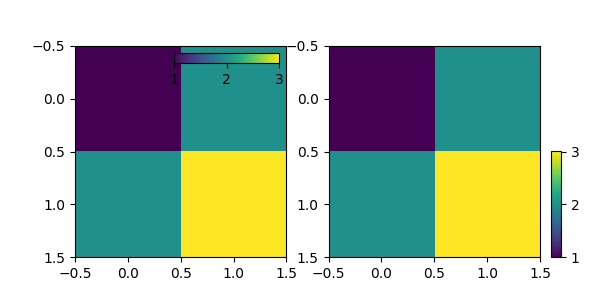# Controlling the position and size of colorbars with Inset Axes#

This example shows how to control the position, height, and width of colorbars using inset_axes.

Inset axes placement is controlled as for legends: either by providing a loc option ("upper right", "best", ...), or by providing a locator with respect to the parent bbox. Parameters such as bbox_to_anchor and borderpad likewise work in the same way, and are also demonstrated here.import matplotlib.pyplot as plt
from mpl_toolkits.axes_grid1.inset_locator import inset_axes

fig, (ax1, ax2) = plt.subplots(1, 2, figsize=[6, 3])

im1 = ax1.imshow([[1, 2], [2, 3]])
axins1 = inset_axes(
ax1,
width="50%",  # width: 50% of parent_bbox width
height="5%",  # height: 5%
loc="upper right",
)
axins1.xaxis.set_ticks_position("bottom")
fig.colorbar(im1, cax=axins1, orientation="horizontal", ticks=[1, 2, 3])

im = ax2.imshow([[1, 2], [2, 3]])
axins = inset_axes(
ax2,
width="5%",  # width: 5% of parent_bbox width
height="50%",  # height: 50%
loc="lower left",
bbox_to_anchor=(1.05, 0., 1, 1),
bbox_transform=ax2.transAxes,